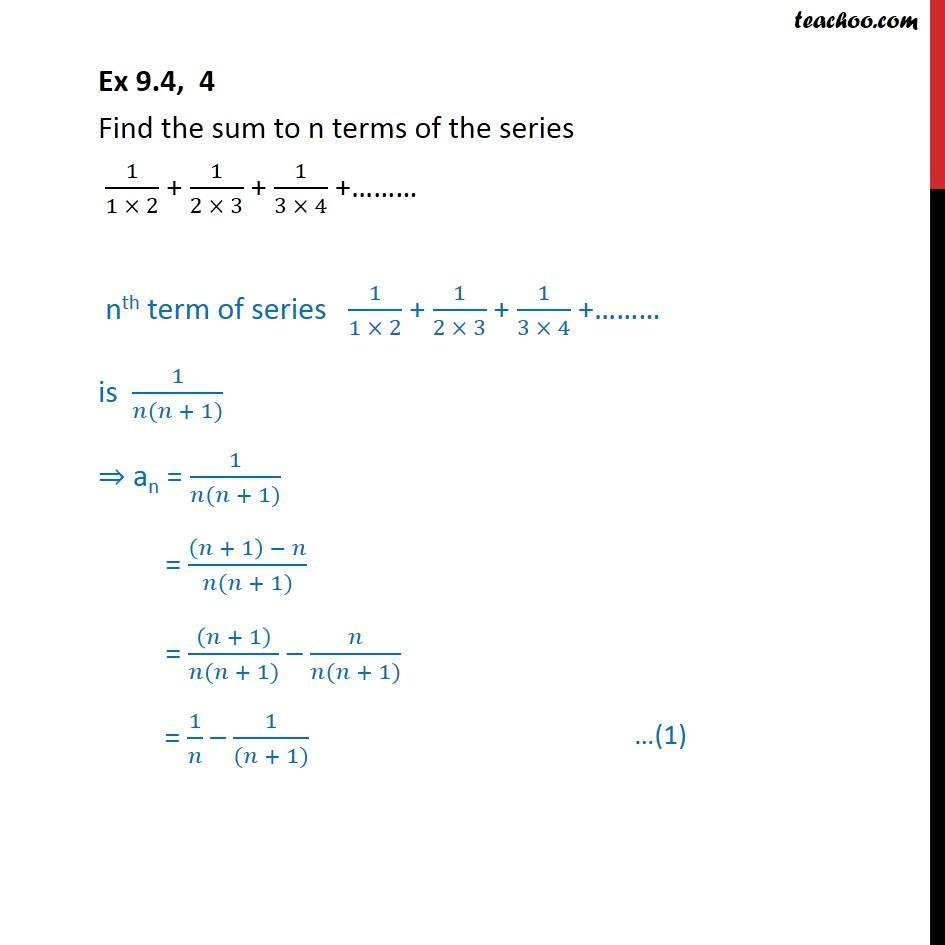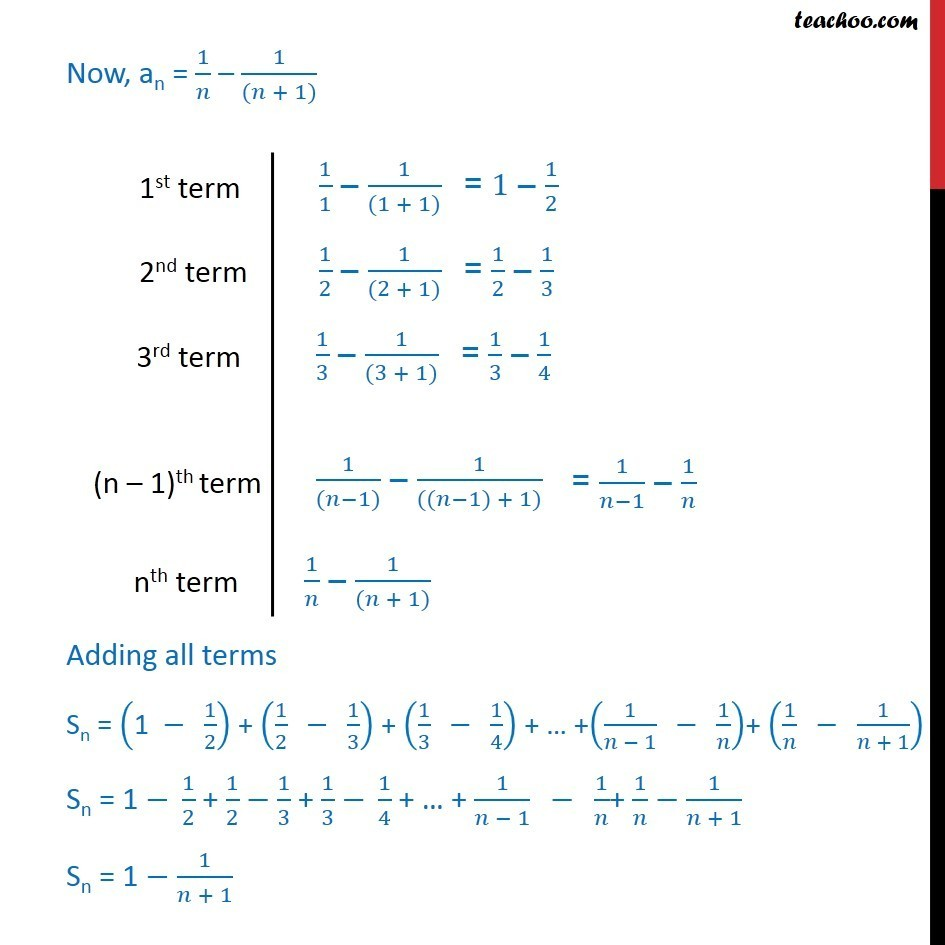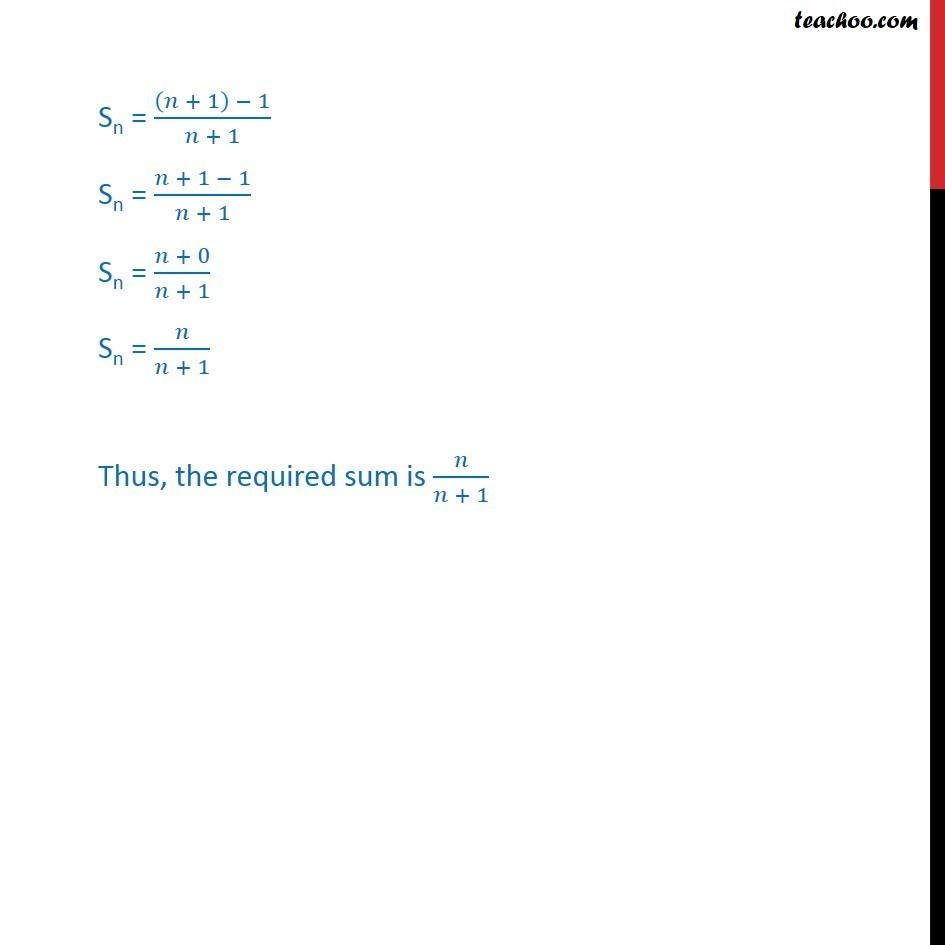Finding sum from nth number

Chapter 8 Class 11 Sequences and Series
Concept wiseLearn in your speed, with individual attention - Teachoo Maths 1-on-1 Class

### Transcript

Question 4 Find the sum to n terms of the series 1/(1 2) + 1/(2 3) + 1/(3 4) + nth term of series 1/(1 2) + 1/(2 3) + 1/(3 4) + is 1/( ( + 1)) an = 1/( ( + 1)) = (( + 1) )/( ( + 1)) = (( + 1))/( ( + 1)) /( ( + 1)) = 1/ 1/(( + 1)) Now, an = 1/ 1/(( + 1)) Adding all terms Sn = ("1 " " " 1/2) + (1/2 " " " " 1/3) + (1/3 " " " " 1/4) + +(1/( 1) " " " " 1/ )+ (1/ " " " " 1/( + 1)) Sn = 1 1/2 + 1/2 1/3 + 1/3 1/4 + + 1/( 1) " " " " 1/ + 1/ 1/( + 1) Sn = 1 1/( + 1) Sn = (( + 1) 1)/( + 1) Sn = ( + 1 1)/( + 1) Sn = ( + 0)/( + 1) Sn = /( + 1) Thus, the required sum is /( + 1)